# Hone advanced Bash skills by building Minesweeper

## The nostalgia of classic games can be a great source for mastering programming. Deep dive into Bash with Minesweeper.Image by :
Opensource.com

## Subscribe now

Get the highlights in your inbox every week.

I am no expert on teaching programming, but when I want to get better at something, I try to find a way to have fun with it. For example, when I wanted to get better at shell scripting, I decided to practice by programming a version of the Minesweeper game in Bash.

If you are an experienced Bash programmer and want to hone your skills while having fun, follow along to write your own version of Minesweeper in the terminal. The complete source code is found in this GitHub repository.

Before I started writing any code, I outlined the ingredients I needed to create my game:

1. Print a minefield
2. Create the gameplay logic
3. Create logic to determine the available minefield
4. Keep count of available and discovered (extracted) mines
5. Create the endgame logic

In Minesweeper, the game world is a 2D array (columns and rows) of concealed cells. Each cell may or may not contain an explosive mine. The player's objective is to reveal cells that contain no mine, and to never reveal a mine. Bash version of the game uses a 10x10 matrix, implemented using simple bash arrays.

First, I assign some random variables. These are the locations that mines could be placed on the board. By limiting the number of locations, it will be easy to build on top of this. The logic could be better, but I wanted to keep the game looking simple and a bit immature. (I wrote this for fun, but I would happily welcome your contributions to make it look better.)

The variables below are some default variables, declared to call randomly for field placement, like the variables a-g, we will use them to calculate our extractable mines:

```# variables
score=0 # will be used to store the score of the game
# variables below will be used to randomly get the extract-able cells/fields from our mine.
a="1 10 -10 -1"
b="-1 0 1"
c="0 1"
d="-1 0 1 -2 -3"
e="1 2 20 21 10 0 -10 -20 -23 -2 -1"
f="1 2 3 35 30 20 22 10 0 -10 -20 -25 -30 -35 -3 -2 -1"
g="1 4 6 9 10 15 20 25 30 -30 -24 -11 -10 -9 -8 -7"
#
# declarations
declare -a room  # declare an array room, it will represent each cell/field of our mine.```

Next, I print my board with columns (0-9) and rows (a-j), forming a 10x10 matrix to serve as the minefield for the game. (M is a 100-value array with indexes 0-99.) If you want to know more about Bash arrays, read You don't know Bash: An introduction to Bash arrays.

Lets call it a function, plough,  we print the header first: two blank lines, the column headings, and a line to outline the top of the playing field:

```printf '\n\n'
printf '%s' "     a   b   c   d   e   f   g   h   i   j"
printf '\n   %s\n' "-----------------------------------------"```

Next, I establish a counter variable, called r, to keep track of how many horizontal rows have been populated. Note that, we will use the same counter variable 'r' as our array index later in the game code. In a Bash for loop, using the seq command to increment from 0 to 9, I print a digit (d%) to represent the row number (\$row, which is defined by seq):

```r=0 # our counter
for row in \$(seq 0 9); do
printf '%d  ' "\$row" # print the row numbers from 0-9```

Before we move ahead from here, lets check what we have made till now. We printed sequence [a-j] horizontally first and then we printed row numbers in a range [0-9], we will be using these two ranges to act as our users input coordinates to locate the mine to extract.

Next, Within each row, there is a column intersection, so it's time to open a new for loop. This one manages each column, so it essentially generates each cell in the playing field. I have added some helper functions that you can see the full definition of in the source code. For each cell,  we need something to make the field look like a mine, so we initialize the empty ones with a dot (.), using a custom function called is_null_field. Also, we need an array variable to store the value for each cell, we will use the predefined global array variable room along with an index variable r. As r increments, we iterate over the cells, dropping mines along the way.

```  for col in \$(seq 0 9); do
((r+=1))  # increment the counter as we move forward in column sequence
is_null_field \$r  # assume a function which will check, if the field is empty, if so, initialize it with a dot(.)
printf '%s \e[33m%s\e[0m ' "|" "\${room[\$r]}" # finally print the separator, note that, the first value of \${room[\$r]} will be '.', as it is just initialized.
#close col loop
done```

Finally, I keep the board well-defined by enclosing the bottom of each row with a line, and then close the row loop:

```printf '%s\n' "|"   # print the line end separator
printf '   %s\n' "-----------------------------------------"
# close row for loop
done
printf '\n\n'```

The full plough function looks like:

```plough()
{
r=0
printf '\n\n'
printf '%s' "     a   b   c   d   e   f   g   h   i   j"
printf '\n   %s\n' "-----------------------------------------"
for row in \$(seq 0 9); do
printf '%d  ' "\$row"
for col in \$(seq 0 9); do
((r+=1))
is_null_field \$r
printf '%s \e[33m%s\e[0m ' "|" "\${room[\$r]}"
done
printf '%s\n' "|"
printf '   %s\n' "-----------------------------------------"
done
printf '\n\n'
}```

It took me some time to decide on needing the is_null_field, so let's take a closer look at what it does. We need a dependable state from the beginning of the game. That choice is arbitrary–it could have been a number or any character. I decided to assume everything was declared as a dot (.) because I believe it makes the gameboard look pretty. Here's what that looks like:

```is_null_field()
{
local e=\$1 # we used index 'r' for array room already, let's call it 'e'
if [[ -z "\${room[\$e]}" ]];then
room[\$r]="."  # this is where we put the dot(.) to initialize the cell/minefield
fi
}```

Now that, I have all the cells in our mine initialized, I get a count of all available mines by declaring and later calling a simple function shown below:

```get_free_fields()
{
free_fields=0    # initialize the variable
for n in \$(seq 1 \${#room[@]}); do
if [[ "\${room[\$n]}" = "." ]]; then  # check if the cells has initial value dot(.), then count it as a free field.
((free_fields+=1))
fi
done
}```

Here is the printed minefield, where [a-j] are columns, and [0-9] are rows.

## minefield.png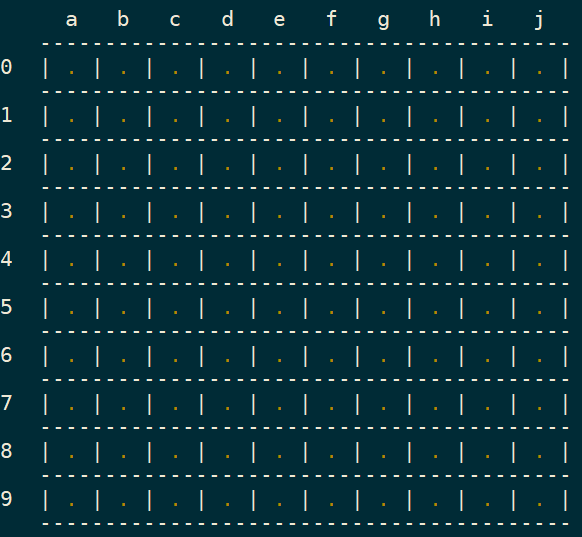## Create the logic to drive the player

The player logic reads an option from stdin as a coordinate to the mines and extracts the exact field on the minefield. It uses Bash's parameter expansion to extract the column and row inputs, then feeds the column to a switch that points to its equivalent integer notation on the board, to understand this, see the values getting assigned to variable 'o' in the switch case statement below. For instance, a player might enter c3, which Bash splits into two characters: c and 3. For simplicity, I'm skipping over how invalid entry is handled.

```  colm=\${opt:0:1}  # get the first char, the alphabet
ro=\${opt:1:1}    # get the second char, the digit
case \$colm in
a ) o=1;;      # finally, convert the alphabet to its equivalent integer notation.
b ) o=2;;
c ) o=3;;
d ) o=4;;
e ) o=5;;
f ) o=6;;
g ) o=7;;
h ) o=8;;
i ) o=9;;
j ) o=10;;
esac```

Then it calculates the exact index and assigns the index of the input coordinates to that field.

There is also a lot of use of shuf command here, shuf is a Linux utility designed to provide a random permutation of information where the -i option denotes indexes or possible ranges to shuffle and -n denotes the maximum number or output given back. Double parentheses allow for mathematical evaluation in Bash, and we will use them heavily here.

Let's assume our previous example received c3 via stdin. Then, ro=3 and o=3 from above switch case statement converted c to its equivalent integer, put it into our formula to calculate final index 'i'.

```  i=\$(((ro*10)+o))   # Follow BODMAS rule, to calculate final index.
is_free_field \$i \$(shuf -i 0-5 -n 1)   # call a custom function that checks if the final index value points to a an empty/free cell/field.```

Walking through this math to understand how the final index 'i' is calculated:

```i=\$(((ro*10)+o))
i=\$(((3*10)+3))=\$((30+3))=33```

The final index value is 33. On our board, printed above, the final index points to 33rd cell and that should be 3rd (starting from 0, otherwise 4th) row and 3rd (C) column.

## Create the logic to determine the available minefield

To extract a mine, after the coordinates are decoded and the index is found, the program checks whether that field is available. If it's not, the program displays a warning, and the player chooses another coordinate.

In this code, a cell is available if it contains a dot (.) character. Assuming it's available, the value in the cell is reset and the score is updated. If a cell is unavailable because it does not contain a dot, then a variable not_allowed is set. For brevity, I leave it to you to look at the source code of the game for the contents of the warning statement in the game logic.

```is_free_field()
{
local f=\$1
local val=\$2
not_allowed=0
if [[ "\${room[\$f]}" = "." ]]; then
room[\$f]=\$val
score=\$((score+val))
else
not_allowed=1
fi
}```

## extractmines.png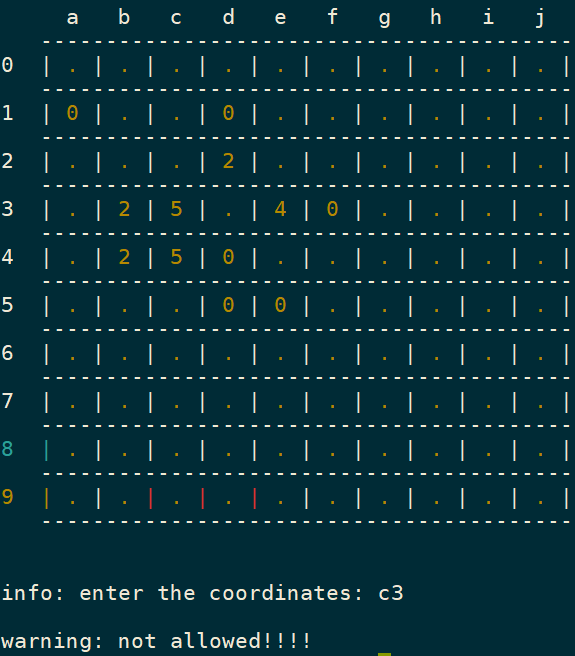If the coordinate entered is available, the mine is discovered, as shown below. When h6 is provided as input, some values at random populated on our minefields, these values are added to users score after the mins are extracted.

## extractmines2.png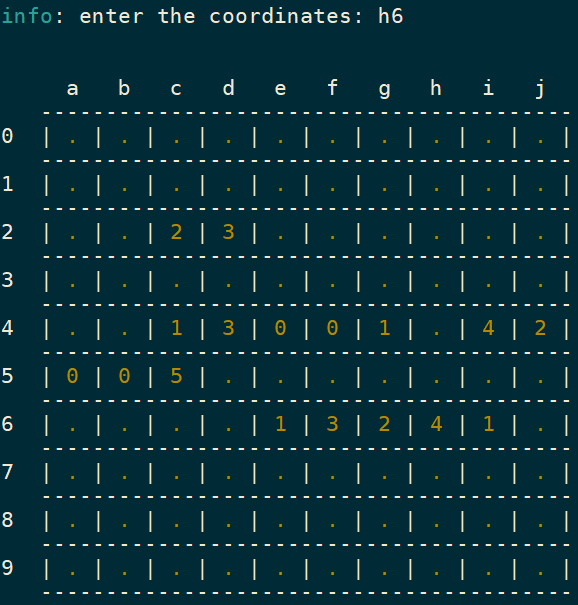Now remember the variables we declared at the start, [a-g], I will now use them here to extract random mines assigning their value to the variable m using Bash indirection. So, depending upon the input coordinates, the program picks a random set of additional numbers (m) to calculate the additional fields to be populated (as shown above) by adding them to the original input coordinates, represented here by i (calculated above).

Please note the character X in below code snippet, is our sole GAME-OVER trigger, we added it to our shuffle list to appear at random, with the beauty of shuf command, it can appear after any number of chances or may not even appear for our lucky winning user.

```m=\$(shuf -e a b c d e f g X -n 1)   # add an extra char X to the shuffle, when m=X, its GAMEOVER
if [[ "\$m" != "X" ]]; then        # X will be our explosive mine(GAME-OVER) trigger
for limit in \${!m}; do          # !m represents the value of value of m
field=\$(shuf -i 0-5 -n 1)     # again get a random number and
index=\$((i+limit))            # add values of m to our index and calculate a new index till m reaches its last element.
is_free_field \$index \$field
done```

I want all revealed cells to be contiguous to the cell selected by the player.

## extractmines3.png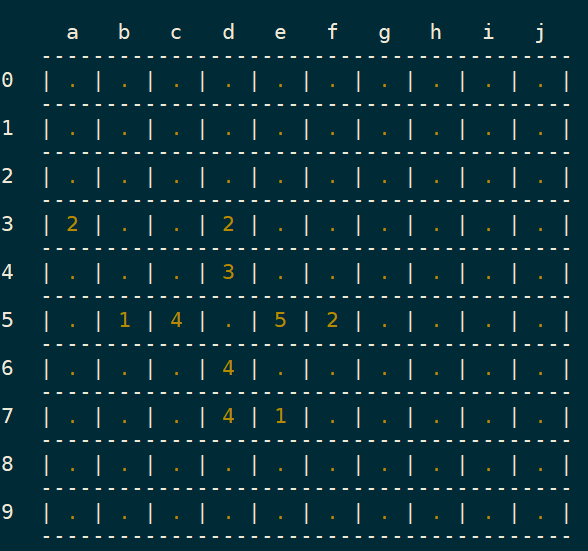## Keep a count of available and extracted mines

The program needs to keep track of available cells in the minefield; otherwise, it keeps asking the player for input even after all the cells have been revealed. To implement this, I create a variable called free_fields, initially setting it to 0. In a for loop defined by the remaining number of available cells/fields in our minefields. If a cell contains a dot (.), then the count of free_fields is incremented.

```get_free_fields()
{
free_fields=0
for n in \$(seq 1 \${#room[@]}); do
if [[ "\${room[\$n]}" = "." ]]; then
((free_fields+=1))
fi
done
}```

Wait, what if, the free_fields=0? That means, our user had extracted all the mines. Please feel free to look at the exact code to understand better.

```if [[ \$free_fields -eq 0 ]]; then   # well that means you extracted all the mines.
printf '\n\n\t%s: %s %d\n\n' "You Win" "you scored" "\$score"
exit 0
fi```

## Create the logic for Gameover

For the Gameover situation, we print to the middle of the terminal using some nifty logic that I leave it to the reader to explore how it works.

```if [[ "\$m" = "X" ]]; then
g=0                      # to use it in parameter expansion
room[\$i]=X               # override the index and print X
for j in {42..49}; do    # in the middle of the minefields,
out="gameover"
k=\${out:\$g:1}          # print one alphabet in each cell
room[\$j]=\${k^^}
((g+=1))
done
fi```

Finally, we can print the two lines which are most awaited.

```if [[ "\$m" = "X" ]]; then
printf '\n\n\t%s: %s %d\n' "GAMEOVER" "you scored" "\$score"
printf '\n\n\t%s\n\n' "You were just \$free_fields mines away."
exit 0
fi```

## gameover.png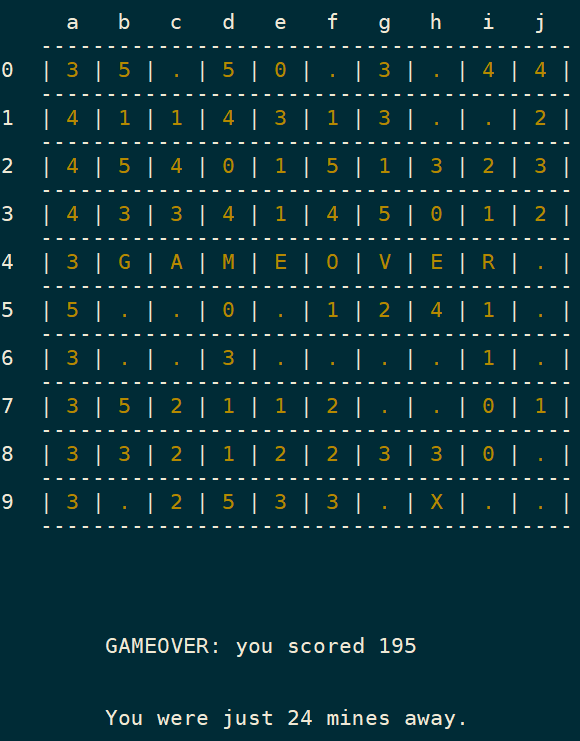That's it, folks! If you want to know more, access the source code for this Minesweeper game and other games in Bash from my GitHub repo. I hope it gives you some inspiration to learn more Bash and to have fun while doing so.